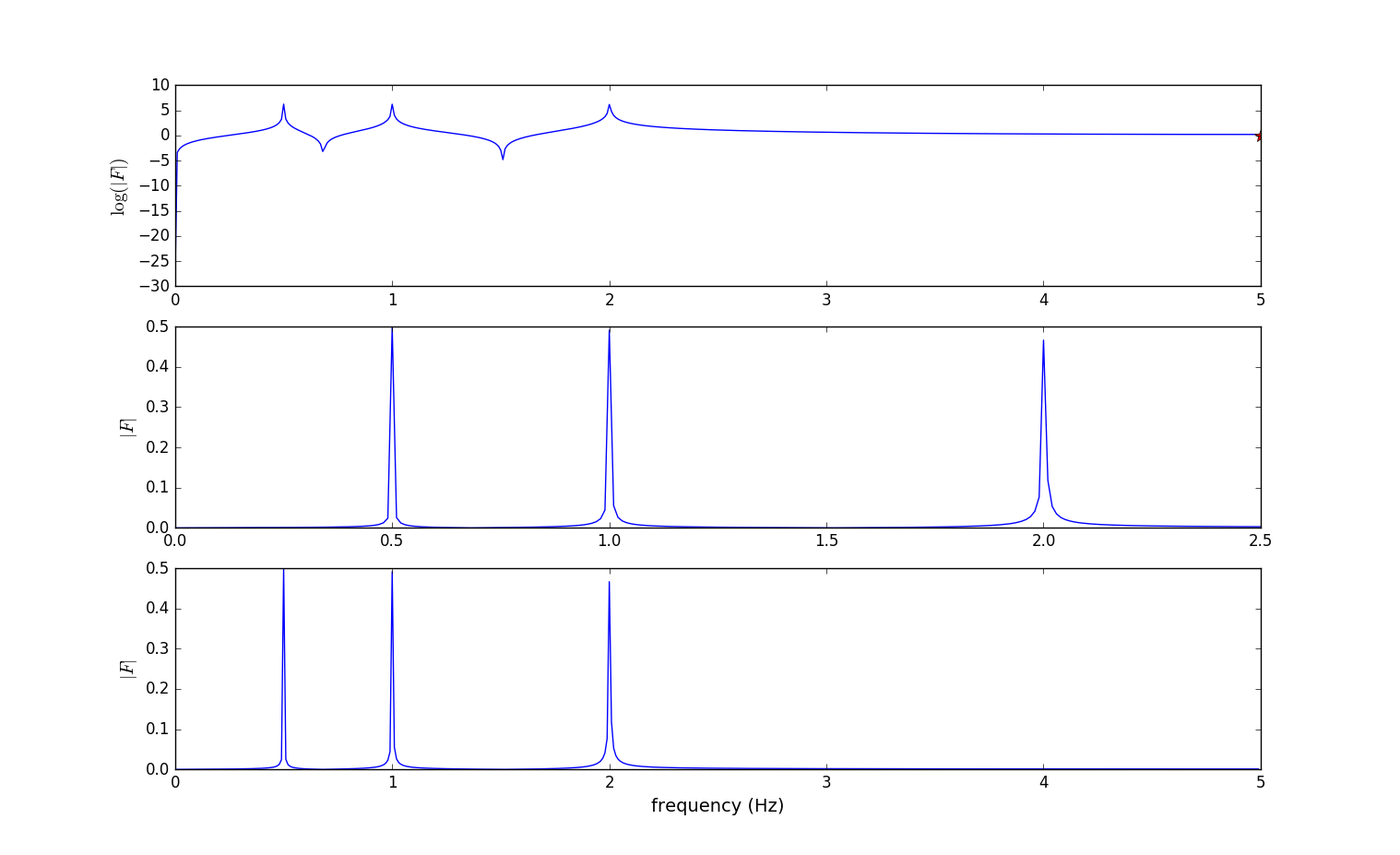# 15. Scipy Tutorial- 快速傅立叶变换fft

import numpy as np
import matplotlib.pyplot as plt
def signal_samples(t):
return np.sin(1 * np.pi * t) + np.sin(2 * np.pi * t) + np.sin(4 * np.pi * t)

B = 5.0
f_s = 2 * B
delta_f = 0.01
N = int(f_s / delta_f)
T = N / f_s
t = np.linspace(0, T, N)
f_t = signal_samples(t)

fig, axes = plt.subplots(1, 2, figsize=(8, 3), sharey=True)
axes.plot(t, f_t)
axes.set_xlabel("time (s)")
axes.set_ylabel("signal")
axes.plot(t, f_t)
axes.set_xlim(0, 5)
axes.set_xlabel("time (s)")
plt.show()

from scipy import fftpack
F = fftpack.fft(f_t)
f = fftpack.fftfreq(N, 1.0/f_s)
fig, axes = plt.subplots(3, 1, figsize=(8, 6))

axes.plot(B, 0, 'r*', markersize=10)
axes.set_ylabel("$\log(|F|)$", fontsize=14)

axes.set_xlim(0, 2.5)
axes.set_ylabel("$|F|$", fontsize=14)

axes.set_xlabel("frequency (Hz)", fontsize=14)
axes.set_ylabel("$|F|$", fontsize=14)
plt.show()


(1). 定义可以生成离散时域信号的函数$signal_samples$：

$$y = \sin(\pi t) +\sin(2\pi t) + \sin(4\pi t)$$ 对应频率分别为$2Hz$、$1Hz$和$0.5Hz$、

import numpy as np
import matplotlib.pyplot as plt
def signal_samples(t):
return np.sin(1 * np.pi * t) + np.sin(2 * np.pi * t) + np.sin(4 * np.pi * t)


(2). 下面定义的是采样率等信息。$\odot$由于定义的产生时域信号的函数signal_samples里的正弦函数的频率最大是$2Hz$所以依据采样定理，采样率大于信号中最高频率成分的2倍，那么$f_s > 2 * 2 = 4$即可，为了可视化化更清晰些，可以设定$\ \ f_s = 10Hz\$。$\odot$设置频谱(frequency spectrum)精度$\Delta f = 0.01Hz$，$\odot$那么需要的采样点的个数$N = \frac{f_s}{\Delta f} = \frac{10}{0.01} = 1000$个。$\odot$需要在$T = \frac{N}{f_s} = \frac{1000}{10} = 100s$秒完成(采用时间)。

B = 5.0
f_s = 2 * B
delta_f = 0.01
N = int(f_s / delta_f)
T = N / f_s


(3). 采样时域信号。$t$表示从$100$秒内共1000个采样点(时间点)。连续信号经采样变成离散信号。

t = np.linspace(0, T, N)
f_t = signal_samples(t)


t传给函数signal_samples生成时域上的信号$f_t$的采样数据共1000个。

(4). 绘制时域信号f_t的可视化图。

t = np.linspace(0, T, N)
f_t = signal_samples(t)
fig, axes = plt.subplots(1, 2, figsize=(8, 3), sharey=True)
axes.plot(t, f_t)
axes.set_xlabel("time (s)")
axes.set_ylabel("signal")
axes.plot(t, f_t)
axes.set_xlim(0, 5)
axes.set_xlabel("time (s)")
plt.show()


(5). 时域信号经fft处理后转化为频率信号数据。scipy的fftpack包里的fft函数可以将采样得到的离散信号从时域转为频域的数据。而fftfreq函数可以求得各个采样点的频率值。

from scipy import fftpack
F = fftpack.fft(f_t)#参数为时域信号
f = fftpack.fftfreq(N, 1.0/f_s)#参数为采样点数和周期


(6). 绘制频率信号可视化图。

fig, axes = plt.subplots(3, 1, figsize=(8, 6))

axes.plot(B, 0, 'r*', markersize=10)
axes.set_ylabel("$\log(|F|)$", fontsize=14)

axes.set_xlim(0, 2.5)
axes.set_ylabel("$|F|$", fontsize=14)

axes.set_xlabel("frequency (Hz)", fontsize=14)
axes.set_ylabel("$|F|$", fontsize=14)
plt.show()可视化图中间的图在频率为$2Hz$、$1Hz$、$0.5Hz$处出现了峰值(peak)对应于函数$signal_samples$里的各个正弦波的频率。

• 加噪声。前面的例子里的信号是干净的信号，这里对$signal_samples$进行简单的处理，增加一点儿噪声，看看fft的用处如何？
def signal_samples(t):
return (2 * np.sin(2 * np.pi * t) + 3 * np.sin(22 * 2 * np.pi * t) + 2 * np.random.randn(*np.shape(t)))


import numpy as np
import matplotlib.pyplot as plt
def signal_samples(t):
return (2 * np.sin(2 * np.pi * t) + 3 * np.sin(22 * 2 * np.pi * t) + 2 * np.random.randn(*np.shape(t)))
B = 30.0
f_s = 2 * B
delta_f = 0.01
N = int(f_s / delta_f)
T = N / f_s
t = np.linspace(0, T, N)
f_t = signal_samples(t)
fig, axes = plt.subplots(1, 2, figsize=(8, 3), sharey=True)
axes.plot(t, f_t)
axes.set_xlabel("time (s)")
axes.set_ylabel("signal")
axes.plot(t, f_t)
axes.set_xlim(0, 5)
axes.set_xlabel("time (s)")
plt.show()

from scipy import fftpack
F = fftpack.fft(f_t)
f = fftpack.fftfreq(N, 1.0/f_s)
fig, axes = plt.subplots(3, 1, figsize=(8, 6))

axes.set_ylabel("$\log(|F|)$", fontsize=14)
axes.set_ylabel("$|F|$", fontsize=14)
axes.set_ylabel("$|F|$", fontsize=14)